# Percentage Worksheet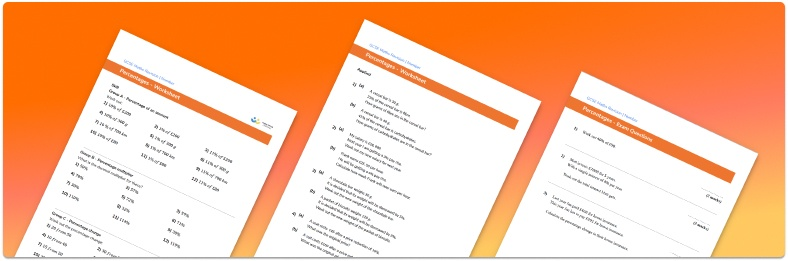• Section 1 of the percentage worksheet contains 36 skills-based percentage questions, in 3 groups to support differentiation
• Section 2 contains 4 applied percentage questions with a mix of percentage word problems and deeper problem solving questions
• Section 3 contains 3 foundation and higher level GCSE exam style percentage questions
• Answer keys and a mark scheme for all percentage questions are provided
• Questions follow variation theory with plenty of opportunities for students to work independently at their own level
• All questions created by fully qualified expert secondary maths teachers
• Suitable for GCSE maths revision for AQA, OCR and Edexcel exam boards
• Other printable percent worksheets are available, including percentage of an amount and percentage multiplier.

• This field is for validation purposes and should be left unchanged.

You can unsubscribe at any time (each email we send will contain an easy way to unsubscribe). To find out more about how we use your data, see our privacy policy.

### Percentage at a glance

A percentage is an amount expressed as a fraction with a denominator of 100. For example 35% means ‘35 out of 100’, 35 hundredths or 35 over 100. In decimal form, we write 0.35. A percentage does not always have to be a whole number.

Basic skills include finding percentages of a given number, converting into fraction or decimal number form, or solving percent problems involving percentage increase or decrease. Percentage calculations are often related to real life – for example, finding the sale price of an item by subtracting the percentage reduction from the original cost.

The topic extends further to work on reverse percentages (finding the original amount given the percentage change and the ‘new’ amount), as well as simple and compound interest.

Looking forward, students can then progress to additional number worksheets, for example aor aFor more teaching and learning support on Number our GCSE maths lessons provide step by step support for all GCSE maths concepts.

## Related worksheets

Percentage Multiplier Worksheet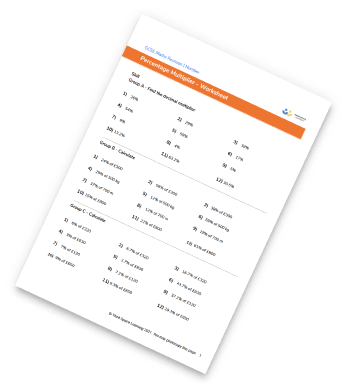Reverse Percentages Worksheet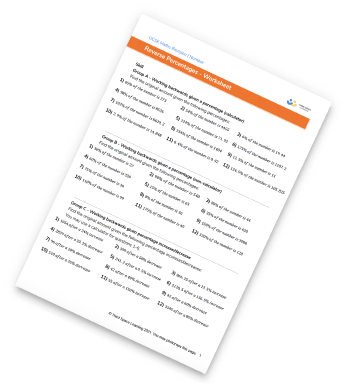One Number As A Percentage Of Another Worksheet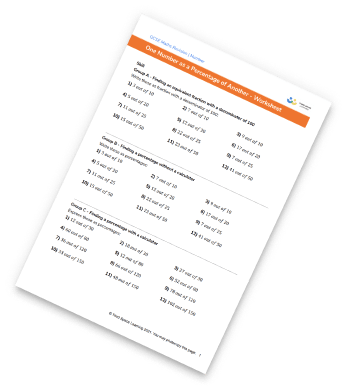Percentage Increase And Decrease Worksheet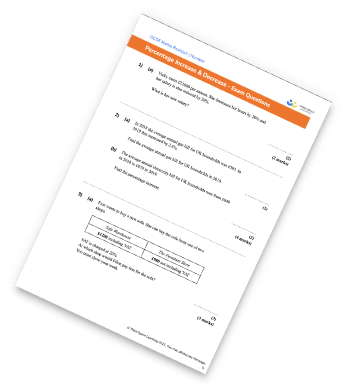## Do you have KS4 students who need more focused attention to succeed at GCSE?There will be students in your class who require individual attention to help them succeed in their maths GCSEs. In a class of 30, it’s not always easy to provide.

Help your students feel confident with exam-style questions and the strategies they’ll need to answer them correctly with our dedicated GCSE maths revision programme.

Lessons are selected to provide support where each student needs it most, and specially-trained GCSE maths tutors adapt the pitch and pace of each lesson. This ensures a personalised revision programme that raises grades and boosts confidence.

Find out more